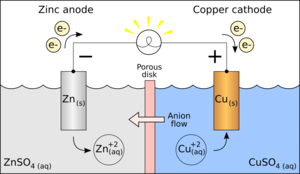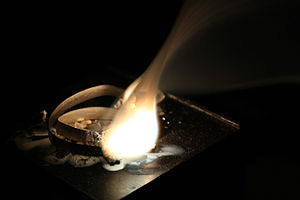# Half-Reactions

$$\newcommand{\vecs}{\overset { \rightharpoonup} {\mathbf{#1}} }$$ $$\newcommand{\vecd}{\overset{-\!-\!\rightharpoonup}{\vphantom{a}\smash {#1}}}$$$$\newcommand{\id}{\mathrm{id}}$$ $$\newcommand{\Span}{\mathrm{span}}$$ $$\newcommand{\kernel}{\mathrm{null}\,}$$ $$\newcommand{\range}{\mathrm{range}\,}$$ $$\newcommand{\RealPart}{\mathrm{Re}}$$ $$\newcommand{\ImaginaryPart}{\mathrm{Im}}$$ $$\newcommand{\Argument}{\mathrm{Arg}}$$ $$\newcommand{\norm}{\| #1 \|}$$ $$\newcommand{\inner}{\langle #1, #2 \rangle}$$ $$\newcommand{\Span}{\mathrm{span}}$$ $$\newcommand{\id}{\mathrm{id}}$$ $$\newcommand{\Span}{\mathrm{span}}$$ $$\newcommand{\kernel}{\mathrm{null}\,}$$ $$\newcommand{\range}{\mathrm{range}\,}$$ $$\newcommand{\RealPart}{\mathrm{Re}}$$ $$\newcommand{\ImaginaryPart}{\mathrm{Im}}$$ $$\newcommand{\Argument}{\mathrm{Arg}}$$ $$\newcommand{\norm}{\| #1 \|}$$ $$\newcommand{\inner}{\langle #1, #2 \rangle}$$ $$\newcommand{\Span}{\mathrm{span}}$$

A half reaction is either the oxidation or reduction reaction component of a redox reaction. A half reaction is obtained by considering the change in oxidation states of individual substances involved in the redox reaction.

Often, the concept of half-reactions is used to describe what occurs in an electrochemical cell, such as a Galvanic cell battery. Half-reactions can be written to describe both the metal undergoing oxidation (known as the anode) and the metal undergoing reduction (known as the cathode).

Half-reactions are often used as a method of balancing redox reactions. For oxidation-reduction reactions in acidic conditions, after balancing the atoms and oxidation numbers, one will need to add H+ ions to balance the hydrogen ions in the half reaction. For oxidation-reduction reactions in basic conditions, after balancing the atoms and oxidation numbers, first treat it as an acidic solution and then add OH- ions to balance the H+ ions in the half reactions (which would give H2O).

## Example: Zn and Cu Galvanic cellGalvanic cell

Consider the Galvanic cell shown in the image to the right: it is constructed with a piece of zinc (Zn) submerged in a solution of zinc sulfate (ZnSO2) and a piece of copper (Cu) submerged in a solution of copper(II) sulfate (CuSO4). The overall reaction is:

Zn(s) + CuSO4(aq) → ZnSO4(aq) + Cu(s)

At the Zn anode, oxidation takes place (the metal loses electrons). This is represented in the following oxidation half-reaction (note that the electrons are on the products side):

Zn(s) → Zn2+ + 2e

At the Cu cathode, reduction takes place (electrons are accepted). This is represented in the following reduction half-reaction (note that the electrons are on the reactants side):

Cu2+ + 2e → Cu(s)

## Example: oxidation of magnesiumPhotograph of a burning magnesium ribbon with very short exposure to obtain oxidation detail.

Consider the example burning of magnesium ribbon (Mg). When magnesium burns, it combines with oxygen (O2) from the air to form magnesium oxide (MgO) according to the following equation:

2Mg(s) + O2(g) → 2MgO(s)

Magnesium oxide is an ionic compound containing Mg2+ and O2- ions whereas Mg(s) and O2(g) are elements with no charges. The Mg(s) with zero charge gains a +2 charge going from the reactant side to product side, and the O2(g) with zero charge gains a -2 charge. This is because when Mg(s) becomes Mg2+, it loses 2 electrons. Since there are 2 Mg on left side, a total of 4 electrons are lost according to the following oxidation half reaction:

2Mg(s) → 2Mg2+ + 4e

On the other hand, O2 was reduced: its oxidation state goes from 0 to -2. Thus, a reduction half-reaction can be written for the O2 as it gains 4 electrons:

O2(g) + 4e → 2O2-

The overall reaction is the sum of both half-reactions:

2Mg(s) + O2(g) + 4e →2Mg2+ + 2O2- + 4e

When chemical reaction, especially, redox reaction takes place, we do not see the electrons as they appear and disappear during the course of the reaction. What we see is the reactants (starting material) and end products. Due to this, electrons appearing on both sides of the equation are canceled. After canceling, the equation is re-written as

2Mg(s) + O2(g) →2Mg2+ + 2O2-

Two ions, positive (Mg2+) and negative (O2-) exist on product side and they combine immediately to form a compound magnesium oxide (MgO) due to their opposite charges (electrostatic attraction). In any given oxidation-reduction reaction, there are two half-reactions – oxidation half- reaction and reduction half-reaction. The sum of these two half-reactions is the oxidation- reduction reaction.

## Half-reaction balancing method

Consider the reaction below:

Cl2 + 2Fe2+ → 2Cl + 2Fe3+

The two elements involved, iron and chlorine, each change oxidation state; iron from +2 to +3, chlorine from 0 to -1. There are then effectively two half-reactions occurring. These changes can be represented in formulas by inserting appropriate electrons into each half-reaction:

Fe2+ → Fe3+ + e
Cl2 + 2e → 2Cl

Given two half-reactions it is possible, with knowledge of appropriate electrode potentials, to arrive at the full (original) reaction the same way. The decomposition of a reaction into half-reactions is key to understanding a variety of chemical processes. For example, in the above reaction, it can be shown that this is a redox reaction in which Fe is oxidised, and Cl is reduced. Note the transfer of electrons from Fe to Cl. Decomposition is also a way to simplify the balancing of a chemical equation. A chemist can atom balance and charge balance one piece of an equation at a time.

For example:

• Fe2+ → Fe3+ + e- becomes 2Fe2+ → 2Fe3+ + 2e-
• is added to Cl2 + 2e- → 2Cl-
• and finally becomes Cl2 + 2Fe2+ → 2Cl- + 2Fe3+

It is also possible and sometimes necessary to consider a half-reaction in either basic or acidic conditions, as there may be an acidic or basic electrolyte in the redox reaction. Due to this electrolyte it may be more difficult to satisfy the balance of both the atoms and charges. This is done by adding H2O, OH-, e-, and or H+ to either side of the reaction until both atoms and charges are balanced.

Consider the half-reaction below:

PbO2 → PbO

OH-, H2O, and e- can be used to balance the charges and atoms in basic conditions.

2e- + H2O + PbO2 → PbO + 2OH-

Again Consider the half-reaction below:

PbO2 → PbO

H+, H2O, and e- can be used to balance the charges and atoms in acidic conditions.

2e- + 2H+ + PbO2 → PbO + H2O

Notice that both sides are both charge balanced and atom balanced.

Often there will be both H+ and OH- present in acidic and basic conditions but that the resulting reaction of the two ions will yield water H2O (shown below):

H+ + OH- → H2O

### Source

http://en.Wikipedia.org/wiki/Half-reaction

Half-Reactions is shared under a CC BY-SA 4.0 license and was authored, remixed, and/or curated by LibreTexts.